# Random stable roommates

 Importance: Medium ✭✭
 Author(s): Mertens, Stephan
 Subject: Graph Theory » Basic Graph Theory » » Matchings
 Keywords: stable marriage stable roommates
 Posted by: mdevos on: February 26th, 2008
Conjecture   The probability that a random instance of the stable roommates problem onpeople admits a solution is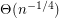.

A system of preferences for a graphis a family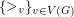so that every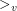is a linear ordering of the neighbors of the vertex. We say thatpreferstoif. A perfect matchinginis stable if there do not exist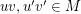so thatprefers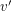toandprefersto.

A famous theorem of Gale-Shapley [GS] proves that every system of preferences on a complete bipartite graphadmits a stable perfect matching. Indeed, they provide an amusing algorithm to construct one. On complete graphs, this problem is known as either the homosexual stable marriage problem, or more commonly, the stable roommate problem. Here there does not always exist a solution (that is, a stable perfect matching), but Irving [I] constructed an algorithm which runs in polynomial time, and outputs a solution if one exists.

Letdenote the probability that a random instance of the stable roommates problem has a solution (so the above conjecture asserts that). The following are the best known asymptotic bounds for(witheven) and hold forsufficiently large. The lower bound is due to Pittel [P] and the upper bound to Pittel and Irving [IP]Mertens [M] did an extensive Monte-Carlo simulation to obtain the above conjecture. Indeed, by guessing at the constant he even offers the stronger conjecture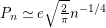.

## Bibliography

[GS] D. Gale D and L. S. Shapley, College admissions and the stability of marriage, Am. Math. Mon. 69 9-15.

[I] R. W. Irving, An efficient algorithm for the stable roommates problem, J. Algorithms 6 577-95.

[IP] B. Pittel and R. W. Irving, An upper bound for the solvability of a random stable roommates instance, Random Struct. Algorithms 5 465-87.

*[M] S. Mertens, Random stable matchings, J. Stat. Mech. Theory Exp. 2005, no. 10 MathSciNet

[P] B. Pittel, The 'stable roommates' problem with random preferences, Ann. Probab. 21 1441-77

* indicates original appearance(s) of problem.